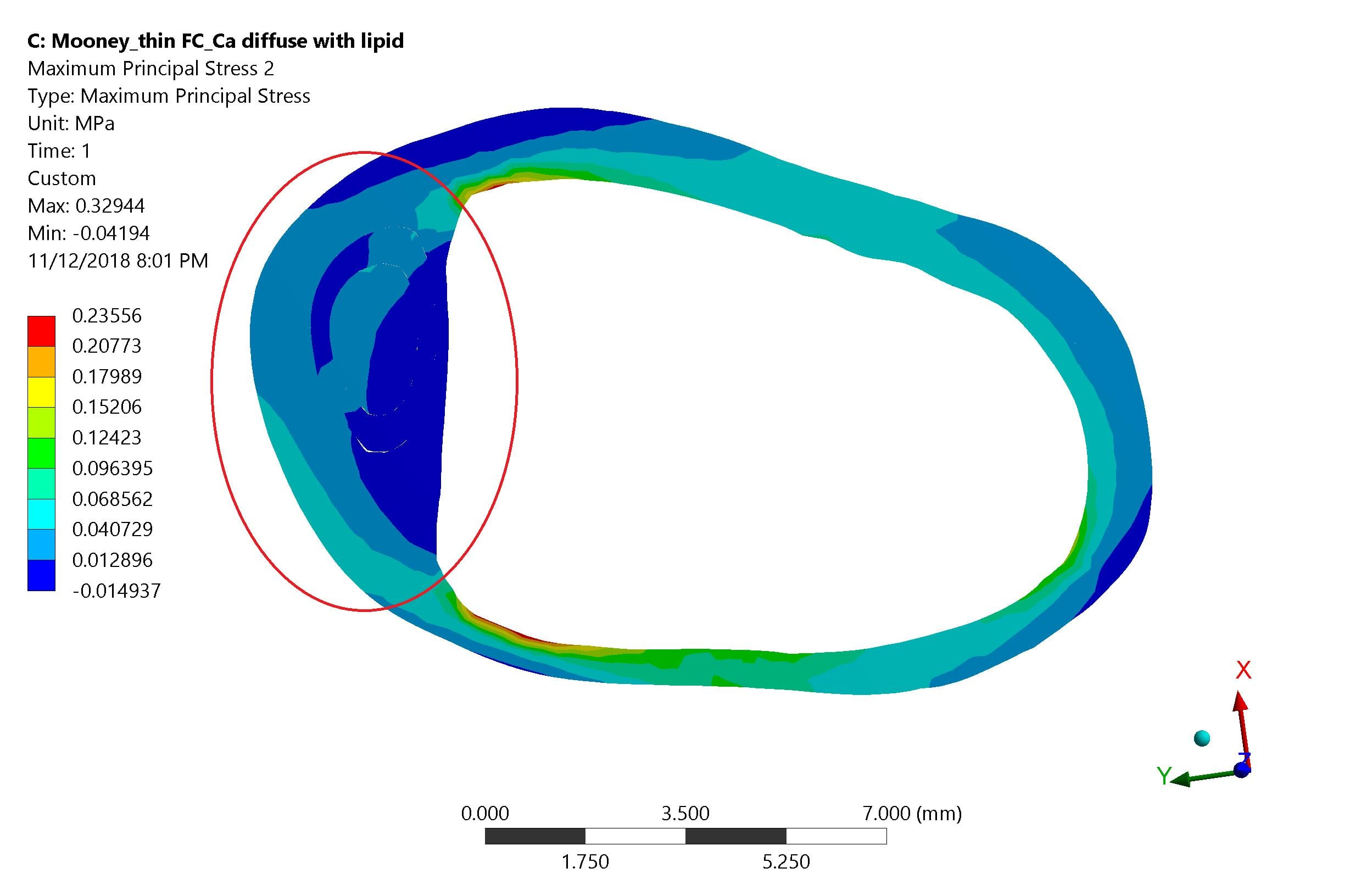## General Mechanical

•Aya.hassan
Subscriber

Hello all,

I have solved a structure model in static structure module. I want to calculate local maximum stress which is the maximum for a defined region of interest in the model. In the next picture, the maximum principal results after I made a cut section. How can I calculate the maximum value of stress or show the results in the area marked with red.•Aniket
Ansys Employee

Hi Aya.hassan,

I can see only one image here, instead of two like you said.

Do you have a mathematical definition of the area? Such as elements which have centroid x coordinate less than 10 mm? If yes, you can create a named selection based on the worksheet for the required elements and then scope the result to the named selection instead of the geometry.

If you don't have a mathematical definition of the area, you can still select the elements by using box select or Lasso select tools and scope the results to the selected elements.